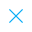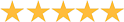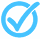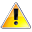Call Support +91-85588-96644

Keep me logged in
You can't leave Captcha Code empty
By submitting this form, you agree to the Terms & Privacy Policy.
ORTests given# Simultaneous Equations and Graphs-2 By TCY All tests

5.0Questions

Time

Highest
score

Level

## ENGLISH

Language

Topics Covered:Linear Equations with One and Two VariablesSimultaneous equations and graphsLinear Equation in 2 VariablesLinear Equations in 2 VariablesLinear Equations in Two VariablesPair of Linear Equations in Two Variables